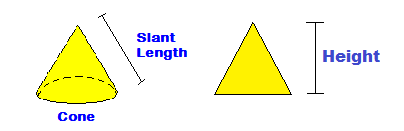CalcuNation

# Cone Area Calculator

## Find the surface area of a cone with this Cone Area Calculator. This calculates the area of the top and the circle area on the bottom of the cone.Height:

Fill in the height and radius of the base to determine the
surface area of a cone.

### How do you find the surface area of a cone?

To find the surface area, use this Cone Area Formula:

(Pi x r2) + (Pi x r x S)

Pi = 3.1416

r = radius of the base

S = the slant length from the base to the tip of the cone
(the slant length is calculated from the height and the radius by
using the pythagorean theorem)

Example: If a cone has a height of 12 inches and a base radius of
4 inches, what is the surface area?

Calculate the slant length = 122 + 42 = S2

S = 12.6491

Cone Area = (3.1416 x 16) + (3.1416 x 4 x 12.6491)

Cone Area = 209.2194 square inches

Other Calculators of Interest:
Circle Circumference Calculator, Sphere Area Calculator, Sphere Volume Calculator

### \$100 Promotion

Win \$100 towards teaching supplies! We want to see your websites and blogs.Enter Here

### Calculator Popups

Scientific Calculator
Simple Calculator

### Calculator Ideas

We use your calculator ideas to create new and useful online calculators.
Submit Calculator Idea